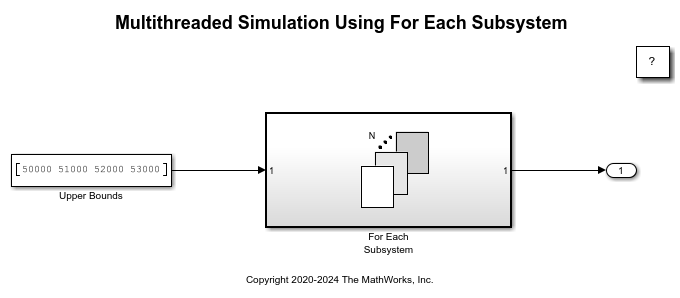# Multithreaded Simulation Using For Each Subsystem

This example shows how to speed up execution of a model on multiple cores using a For Each subsystem in Rapid Accelerator simulation mode.

### Measure the Single-Threaded Simulation Time

In this model, a For Each subsystem accepts a numeric array input. Each element of the array serves as an upper bound in each iteration and feeds into a computationally-intensive algorithm represented by the MATLAB Function block inside the subsystem. The algorithm implemented in the MATLAB Function block computes the largest prime number within a given range using a brute force method, which is for illustrative purposes only.

```modelName = 'slexForEachMultithreaded'; open_system(modelName); ```By default, the multithreaded simulation support for the For Each subsystem is enabled. To opt out of multithreaded simulation, issue a `set_param` command in the MATLAB® Command Window. In a single-threaded simulation, the computations in different iterations of the For Each subsystem are executed sequentially during each time step.

```set_param(modelName, 'MultithreadedSim', 'off'); ```

Build the Rapid Accelerator target for the model. The progress of the code generation and build process is displayed in the MATLAB Command Window. To suppress this display, wrap the command inside `evalc`.

```evalc('Simulink.BlockDiagram.buildRapidAcceleratorTarget(modelName)'); ```

This build step is optional because Simulink® automatically generates the Rapid Accelerator simulation target the first time you simulate the model. In the example, this step serves to separate the overhead in generating the Rapid Accelerator target from the actual model execution time.

Once the Rapid Accelerator target is generated, simulate the model and measure the simulation time using `tic` and `toc`.

```tic evalc('sim(modelName)'); toc ```
```Elapsed time is 61.810143 seconds. ```

### Use Multithreaded Simulation for Speedup on Multiple Cores

To explicitly opt in to multithreading support, issue a `set_param` command in the MATLAB Command Window. In a multithreaded simulation, the computations in different iterations of the For Each subsystem are assigned to multiple cores and executed in parallel for speedup.

```set_param(modelName, 'MultithreadedSim', 'auto'); ```

Build the Rapid Accelerator target again, since the model has been reconfigured.

```evalc('Simulink.BlockDiagram.buildRapidAcceleratorTarget(modelName)'); ```

Simulate the model again and measure the multithreaded simulation time. Notice that the model simulation runs over two times faster than the single-threaded simulation on a machine with four or more cores. Remember that operating system context-switching in multithreaded simulations always results in extra overhead.

```tic evalc('sim(modelName)'); toc ```
```Elapsed time is 27.171541 seconds. ```

### Clean Up

Close the model and remove the generated files.

```bdclose(modelName); ```